Search IntMath
Close

450+ Math Lessons written by Math Professors and Teachers

5 Million+ Students Helped Each Year

1200+ Articles Written by Math Educators and Enthusiasts

Simplifying and Teaching Math for Over 23 Years

# IntMath Newsletter: Fundamental theorem, information paradox

By Murray Bourne, 29 Apr 2015

29 Apr 2015

1. Fundamental Theorem of Calculus
2. Tutor-Web & Khan Academy
3. The Information Paradox
4. Math puzzles
5. Math movie: Accuracy vs precision
6. Final thought - Learned helplessness?

## 1. Fundamental Theorem of Calculus

A reader was looking for some information on the Fundamental Theorem of Calculus. It's a topic I'd been meaning to add to IntMath for some time, so I wrote a page.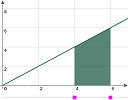The Fundamental Theorem of Calculus ties together the key ideas of differentiation and integration. It includes an applet where you can explore the concepts involved. The Fundamental Theorem of Calculus

## 2. Resource: Tutor-Web

You may be interested to check out Tutor-Web, a "research-based learning environment for personalised education".

Currently topics are available in:

• Mathematics
• Statistics
• Computer Science
• Fishery Science

The idea is to provide free learning help by allocating learning materials and questions which are "just right" for the student. This ensures motivation continues, since students are constantly provided with the right amount of challenge.

Here's a ressearch thesis on Tutor-Web by Anna Helga Jónsdóttir, outlining the development of the system and various experiments with real students.

In many ways it's similar to Khan Academy.

Have any of you tried either system? Let us know in the comments whether you found them useful.

## 3. The Information ParadoxThe Information Paradox is an interesting challenge to the accepted notions of how life on Earth came to be. There's some mathematics involved, too! The Information Paradox

## 4. Math puzzles

The puzzle in the last IntMath Newsletter asked about 3 points on the co-ordinate plane.

Correct answers with explanation were given by Christian, Guido, Darrell, Yousif, Charles, Joseph, Thomas, Grant, and Amin & Nicole.

The variety of approaches used was really interesting, ranging from trigonometric and determinants, to algebraic, perpendicular distance between point and line, area of a triangle and cross product of vectors. If I was to award a prize for the most simple and elegant (recalling Occam's Razor), it would be to Christian and Amin & Nicole, who both used the approach of subtracting known (and unknown) triangle areas from the area of the (known) surrounding rectangle.

Communicating math on the Web

In Michael's answer, he says:

Any chance you could incorporate LaTeX in the comments sections? Would be super cool!

I agree! Actually, it's been available all along.

One of the issues surrounding learning math online is that it is hard to communicate equations and diagrams (in forums, blog comments, or via email).

Joseph's reply involved determinants, and he used spaces to align the answer. It went all over the place so I added <pre> tags so it was more readable. But there are easier ways.

I've had WP Math Publisher on SquareCirclez all along, but it was not very satisfactory.

I've recently set up 2 better alternatives.

(a) You can use simple input to create math in your reply, by entering (for example)

c=sqrt{a^2+b^2}

(b) You can also enter LaTeX. The syntax you use is:

\­( c=\sqrt{a^2+b^2} \­)

The output in both cases will be: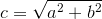I've posted a note near the comment box about these options.

(c) Another option is to send me a mail using the Send math in emails facility. This is better because you get instant feedback on how your math will look. It accepts plain text input (very easy). The syntax to use is here. It's easy for me to add your solution to the blog from there.

### New math puzzle

What is the ratio of the surface area of the tetrahedron UPRW to the surface area of the cube?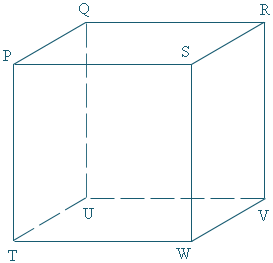Leave your responses here.

### Cheryl's birthday problem

You probably heard about the storm created when the "Cheryl Birthday problem" went viral recently.

Initially the rumor was incorrect (that it was a Singapore primary-level school question, meant for 10- or 11-year olds). It was actually a Math Olympiad question, presented towards the end of a contest and it was "a difficult question meant to sift out the better students".

You can see the question and official solution here.

## 5. Math movie - Accuracy vs precisionThere's often confusion regarding the difference between accuracy and precision. This animation (and following lesson) from TED-Ed gives some insights. What's the difference between accuracy and precision?

TED-Ed is a platform where teachers can create a lesson basesd on any YouTube video. It's a great idea for flipped math classrooms!

## 6. Final thought - Learned helplessness?

IntMath readers can provide feedback or ask questions on each page in IntMath. I've ranted before about some of the impossible questions I've received in the past.

On the feedback page, I have the statement:

Show your working so I can see where you are getting stuck. If you cannot get started, please indicate which part of the question you don't understand.

I follow that up with this:

I won't do the whole problem for you since that only helps you to get a good mark − it doesn't help your learning.

Despite the above statements, the majority of requests I get just contain the question (often missing some vital information). There is no attempt to have a go at the problem (which gives me a better idea how I can help), or to indicate what they have read, and which parts they find confusing. Often, it's usually just the question.

For example, here's one I received this week, which didn't even have a specific question. It just said:

now i m CA form pakistan can u halp me

This gives me no idea where the student is having trouble, and "CA form" doesn't mean much outside of his country (or is it "CA from"?).

I usually write back giving them a hint on how to start their question (usually a link to a page with similar questions), and that's usually the end of the discourse. (Rarely do such people show any sort of gratitude.)

It seems to be a case of learned helplessness. One of the characteristics we should encourage amongst students (and our own children) is resilience, and a desire to plug away at something until they get it. By providing the answer straight away (as a lot of teachers do), students don't get to develop the skill set required to solve the problem. They also don't get much experience in asking good questions about their struggles with a particular concept.

Sometimes, the best way for learners to grow is if we pull back and give them an opportunity to struggle a bit. By clarifying their difficulties, it helps their thought processes. Of course, we need to let them know we are not being nasty, rather we are helping them beyond measure.

Here is an example of a student who puts in the requisite amount of thinking: Summation Notation Question.

Until next time, enjoy whatever you learn.

See the 9 Comments below.

### 9 Comments on “IntMath Newsletter: Fundamental theorem, information paradox”

1. Jaff Lawrence Asuiyi says:

Area of tetrahedron UPRW
=Area(UPW)+Area(UPR)+Area(UWR)+Area(PWR)
=(1/2)[abs((1,-1,1)*(1,-1,0)]+(1/2)[abs((1,-1,1)*(1,0,1)]
+(1/2)[abs((1,0,1)*(1,-1,0)]+(1/2)[abs((-1,0,0)*(-1,-1,0)]
=(1/2)[abs(1,1,2)]+(1/2)[abs(-1,0,1)]+(1/2)[abs(1,1,-1)]
+(1/2)[abs(0,0,1)]
=(1/2)[sqrt(6)+sqrt(2)+sqrt(3)+1]
Area of cube=6*1*1=6
Ratio of area of tetrahedron to area of cube
=(1/2)[sqrt(6)+sqrt(2)+sqrt(3)+1]:6
==[sqrt(6)+sqrt(2)+sqrt(3)+1]:12

2. Sachin Munshi says:

Let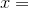edge length of cube.

Let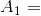area of cube.

Clearly,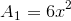. But we know that for the (regular) tetrahedron, the edge lengths are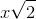.

Letting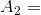area of tetrahedron, it follows that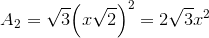Therefore, the ratio of the surface area of the tetrahedron to the surface area of this cube is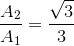.

3. Charles Kibasa says:

FOR THE CURRENT PUZZLE:
The vertices of the given cube are at P, Q, R, S, T, U, V, W, T.
The tetrahedron in it has vertices at U, P, R, W.
Taking a side of the cube to be unity long, the side of the tetrahedron is square root of 2 long by Pythagoras theorem, because it is a diagonal of the face of the cube. The faces of the cube are 6, each 1 square unit area and the faces of the tetrahedron are 4 with each 1/2 square root 3 square units of area. So total surface area of the cube is 6 square units and that of the tetrahedron is 2 times square root 3.
Then the ratio of the surface areas, tetrahedron to cube after calculations is 1/3 square root 3(or (sqrt3)^-1).
Regards.
Charles.

4. Adam C.Rinaldi says:

First, I am 71. I use Khan Academy to learn higher level math and I find it is very good most of the time. There have been a few times where I had to go to other sites to get a better explaination of what he is trying to convey. How would I rate Khan Academy? Very Good!

5. Geeta says:

It was very informative and interesting newsletter.I liked your comment'I won't do the whole problem for you since that only helps you to get a good mark − it doesn't help your learning'.But in most of the typical math teaching teachers don't allow the students think by themselves.

6. Jaff Lawrence Asuiyi says:

Comment on the correct answer to the Maths Puzzle of April, 2015
From what Sachin and Charles have done, I can now figure out the that the tetrahedron in question is regular.
I am very grateful with the way Sachin has presented the solution.
My regards to all those who are contributing to this Newsletter.
Jaff

7. Charles Kibasa says:

Congratulations Sachin for the smart and clear mathematical presentation of the solution to the puzzle. The puzzle was good and well set for activating imagination.
Regards.
Charles.

8. Gyorgy says:

What is a regular tetrahedron, and is this tetrahedron actually regular? Seems like only one face is an equilateral triangle, and the other 3 are isosceles?
[3 + sqrt(3)]/12 ?

9. Gyorgy says:

Never mind. I think I see my mistake now?
Thanks

### Comment Preview

HTML: You can use simple tags like <b>, <a href="...">, etc.

To enter math, you can can either:

1. Use simple calculator-like input in the following format (surround your math in backticks, or qq on tablet or phone):
a^2 = sqrt(b^2 + c^2)
(See more on ASCIIMath syntax); or
2. Use simple LaTeX in the following format. Surround your math with $$ and $$.
$$\int g dx = \sqrt{\frac{a}{b}}$$
(This is standard simple LaTeX.)

NOTE: You can mix both types of math entry in your comment.